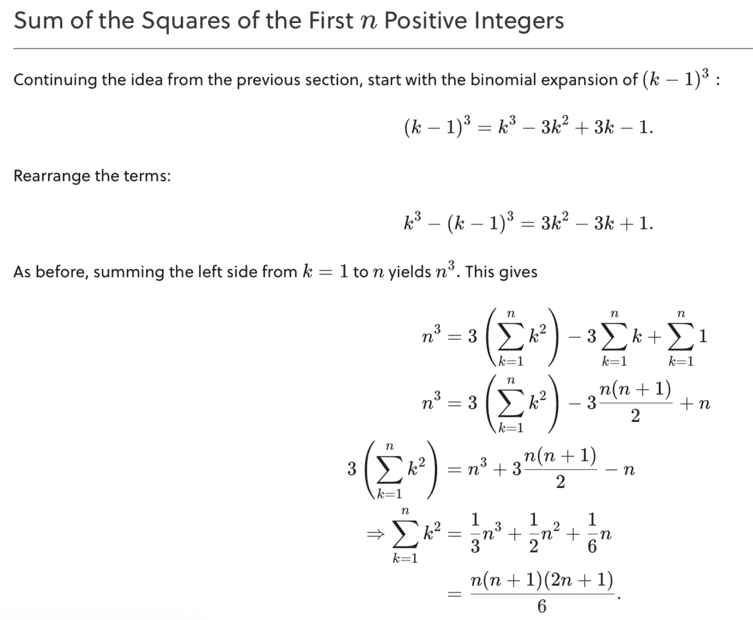# Trying to find an easier way to compute the double sum

Gold Member
Homework Statement:
.
Relevant Equations:
.
I computed the double sum
$$\sum_{i=0}^n\sum_{j=i+1}^n j = \sum_{i=0}^n\big(\frac{n(n+1)}{2}-\frac{i(i+1)}{2}\big)=\frac{n(n+1)(2n+1)}{6}$$
and realized the double sum is equal to $$\sum_{i=1}^ni^2$$
$$\sum_{i=0}^n\sum_{j=i+1}^n j = \sum_{i=1}^ni^2$$

Is there a proof of this equality that doesn't require computing out the sums?

I thought that I should try changing the boundaries of integration

##0\leq i+1\leq j\leq n## so

$$\sum_{i=0}^n\sum_{j=i+1}^n j = \sum_{j=0}^n\sum_{i=0}^j j = \sum_{j=0}^n j(j+1)=\Big(\frac{n(n+1)}{2}+1\Big)\Big(\frac{n(n+1)}{2}\Big)$$

but I was unable to even do this properly since the above isn't equal to ##\frac{n(n+1)(2n+1)}{6}##

Last edited:

Mark44
Mentor
I thought that I should try changing the boundaries of integration
Summation, not integration...
docnet said:
##0\leq i+1\leq j\leq n## so

$$\sum_{i=0}^n\sum_{j=i+1}^n j = \sum_{j=0}^n\sum_{i=0}^j j \dots$$

but I was unable to even do this properly since the above isn't equal to ##\frac{n(n+1)(2n+1)}{6}##
I would work with the two summations above, with paper and pencil, to see if they are generating the same terms. If not, that's your answer.

ergospherical
Gold Member
If I understand correctly, write\begin{align*}
\sum_{i=0}^n \sum_{j=i+1}^n j &= \sum_{i=0}^n \left( \frac{n(n+1)}{2} - \frac{i(i+1)}{2} \right) \\
&= \frac{n(n+1)^2}{2} - \dfrac{1}{2} \sum_{i=0}^n i^2 - \dfrac{1}{2} \sum_{i=0}^n i \\
&= \frac{n(n+1)^2}{2} - \dfrac{1}{2} \sum_{i=0}^n i^2 - \dfrac{n(n+1)}{4} \\
&= \dfrac{3}{2} \cdot \dfrac{n(n+1)(2n+1)}{6} - \dfrac{1}{2} \sum_{i=0}^n i^2
\end{align*}Can you finish it?

Gold Member
If I understand correctly, write\begin{align*}
\sum_{i=0}^n \sum_{j=i+1}^n j &= \sum_{i=0}^n \left( \frac{n(n+1)}{2} - \frac{i(i+1)}{2} \right) \\
&= \frac{n(n+1)^2}{2} - \dfrac{1}{2} \sum_{i=0}^n i^2 - \dfrac{1}{2} \sum_{i=0}^n i \\
&= \frac{n(n+1)^2}{2} - \dfrac{1}{2} \sum_{i=0}^n i^2 - \dfrac{n(n+1)}{4} \\
&= \dfrac{3}{2} \cdot \dfrac{n(n+1)(2n+1)}{6} - \dfrac{1}{2} \sum_{i=0}^n i^2
\end{align*}Can you finish it?
Hi, Thank you so much!

This is the way I computed the sum initially.

$$\dfrac{3}{2} \cdot \dfrac{n(n+1)(2n+1)}{6} - \dfrac{1}{2} \sum_{i=0}^n i^2$$

I used the identity
$$\sum_{i=0}^n i^2=\dfrac{n(n+1)(2n+1)}{6}$$

so
$$\dfrac{3}{2} \cdot \dfrac{n(n+1)(2n+1)}{6} - \dfrac{1}{2} \sum_{i=0}^n i^2=\dfrac{n(n+1)(2n+1)}{6}$$

In the OP I was wondering if this was a coincidence that the double sum was equal to
$$\sum_{i=0}^n i^2$$

sorry, I suck at syntax.

Last edited:
Gold Member
this is the explanation I could find for the sum of squares: In elementary algebra, the binomial theorem (or binomial expansion) describes the algebraic expansion of powers of a binomial.•Office_Shredder
mjc123
Homework Helper
The double sum is equal to
1 + 2 + 3 + 4 + ... + n
+ 2 + 3 + 4 + ... + n
+ 3 + 4 + ... + n
...
+n
=1*1 + 2*2 + 3*3 + ... + n*n

•docnet
Gold Member
The double sum is equal to
1 + 2 + 3 + 4 + ... + n
+ 2 + 3 + 4 + ... + n
+ 3 + 4 + ... + n
...
+n
=1*1 + 2*2 + 3*3 + ... + n*n
well that answers my wuestion nicely. thank you :)

Office_Shredder
Staff Emeritus
•